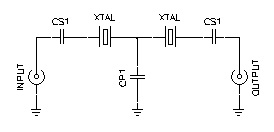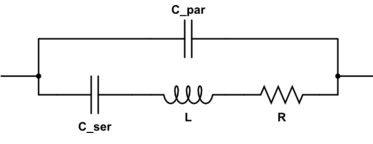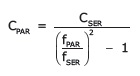#### Question 1—A divider is a logic module that takes two binary numbers and produces their numerical quotient (and optionally, the remainder). The basic structure is a series of subtractions and multiplexers, where the multiplexer uses the result of the subtraciton to select the value that gets passed to the next step. The quotient is formed from the bits used to control the multiplexers, and the remainder is the result of the last subtraction.

If it is implemented purely combinatorially, then the critical path through all of this logic is quite long (even with carry-lookahead in the subtractors) and the clock cycle must be very slow. What could be done to shorten the clock period without losing the ability to get a result on every clock?

#### Answer 1—Pretty much any large chunk of combinatorial logic can be pipelined in order to reduce the clock period. This allows it to produce more results in a given amount of time, at the expense of increasing the latency for any particular result.

Divider logic is very easy to pipeline, and the number of pipeline stages you can use is fairly arbitrary. You could insert a pipeline register after each subtract-mux pair, or you might choose to do two or more subtract-mux stages per pipeline register You could even go so far as to pipeline the subtracts and the muxes separately (or even pipeline *within* each subtract) in order to get the fastest possible clock speed, but this would be rather extreme.

The more pipeline registers you use, the shorter the critical path (and the clock period) can be, but you use more resources (the registers). Also, the overall latency goes up, since you need to account for the setup and propagation times of the pipeline registers in the clock period (in addition to the subtract-mux logic delays). This gets multiplied by the number of pipeline stages in order to compute the total latency.

#### Answer 2—If you don’t need the level of performance provided by a pipelined divider, you can computes the quotient serially, one bit at a time. You would just need one subtractor and one multiplexer, along with registers to hold the input values, quotient bits and the intermediate result.

You could potentially compute more than one bit per clock period using additional subtract-mux stages. This gives you the flexibility to trade off space and time as needed for a particular application.

Question 3—An engineer wanted to build an 8-MHz filter that had a very narrow bandwidth, so he used a crystal lattice filter like this:

####However, when he built and tested his filter, he discovered that while it worked fine around 8 MHz, the attenuation at very high frequencies (e.g., >80 MHz) was very much reduced. What caused this?

#### Answer 3—The equivalent circuit for a quartz crystal is something like this:The components across the bottom represent the mechanical resonance of the crystal itself, while the capacitor at the top represents the capacitance of the electrodes and holder. Typical values are:

• Cser: 10s of fF (yes, femtofarads, 10-15F)
• L: 10s of mH
• R: 10s of ohms
• Cpar: 10s of pF

The crystal has a series-resonant frequency based on just Cser and L. It has a relatively low impedance (basically just R) at this frequency.

It also has a parallel-resonant (sometimes called “antiresonant”) frequency when you consider the entire loop, including Cpar. Since Cser and Cpar are essentially in series, together they have a slightly lower capacitance than Cser alone, so the parallel-resonant frequency is slightly higher. The crystal’s impedance is very high at this frequency.

But at frequencies much higher than either of the resonant frequencies, you can see that the impedance of Cparalone dominates, and this just keeps decreasing with increasing frequency. This reduces the crystal lattice filter to a simple capacitive divider, which passes high freqeuncies with little attenuation.

#### Finally, calculate the value of Cpar required to give that value of capacitance when in series with Cser:Note that all three equations can be combined into one, and this reduces to:Keep up-to-date with our FREE Weekly Newsletter! Don't miss out on upcoming issues of Circuit Cellar. Subscribe to Circuit Cellar Magazine Note: We’ve made the May 2020 issue of Circuit Cellar available as a free sample issue. In it, you’ll find a rich variety of the kinds of articles and information that exemplify a typical issue of the current magazine. Would you like to write for Circuit Cellar? We are always accepting articles/posts from the technical community. Get in touch with us and let's discuss your ideas.